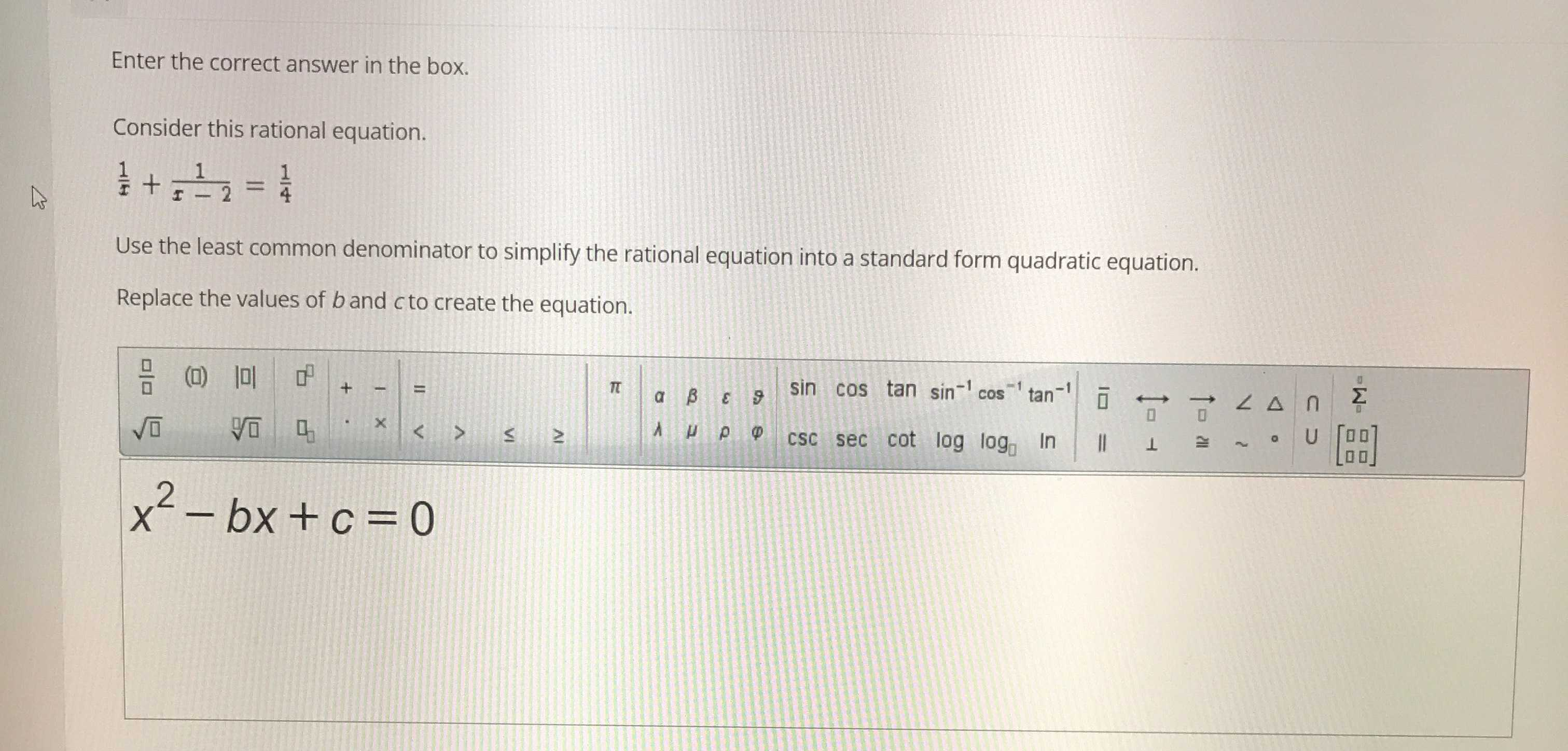### Still have math questions?

Algebra
QuestionEnter the correct answer in the box. Consider this rational equation.

$$\frac { 1 } { x } + \frac { 1 } { x - 2 } = \frac { 1 } { 4 }$$

Use the least common denominator to simplify the rational equation into a standard form quadratic equation. Replace the values of $$b$$ and $$c$$ to create the equation.

$$x ^ { 2 } - b x + c = 0$$

$$x^{2}- 10x+ 8= 0$$